Answer the questions on the basis of the inf...

### Related TestAnswer the questions on the basis of the information given below:
A, B, C. D and E are five brothers. They have assets in the form of flats and cash only. Together they have 9 flats and each brother has at least 1 flat. Value (in terms of rupees) of all flats is equal and constant. Cash with each brother is an integral multiple of Rs. 10 lakhs. The amount of cash with B is just enough to buy one more flat, all the other brothers have cashless than that of B. E has got the maximum number of flats. A number of flats with A is equal to the sum of the number of flats with B and C. Amount of cash with B is double of that with D, but D has got assets worth Rs. 20 lakhs more than that of B.
What is the ratio of the maximum possible value of assets of E to the minimum possible value of assets of C?
• a)
5 : 2
• b)
4 : 1
• c)
2 : 1
• d)
3 : 1Kunaal SatijaE has got the maximum number of flats and number of flats with A is equal to the sum of a number of flats with B and C. As there are 9 flats, so possible solutions are when E, A, B, C and D have 3, 2, 1, 1 and 2 or 4, 2, 1. 1 and 1 flats respectively.
Case I:B and D have one flat and two flats respectively.
Let the value of a flat be Rs. x. So, the amount of cash with B = x.
So total assets of B = 2x
Total assets of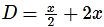As D has got assets worth 20 lakhs more than that of B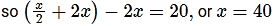Case II:
B and D have one flat each.
Let value of a flat be Rs. x. So, amount of cash with B = x
⇒ Total assets of B = 2x
Total assets of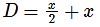As D has got assets worth 20 lakhs more than B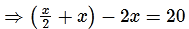Here the value of x is coming as negative. So this case is not possible.
We can collate the final distribution of assets in the following table: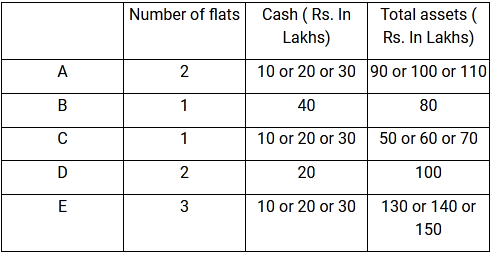Maximum possible assets of E = 150 lakhs and minimum possible assets of C = 50 lakhs. so, Ratio is = 3 : 1​.View courses related to this question Explore CAT courses
 Explore CAT coursesView courses related to this question1 Crore+ students have signed up on EduRev. Have you?

• ### Group QuestionAnswer the following question based on the information given ... more(Scan QR code)